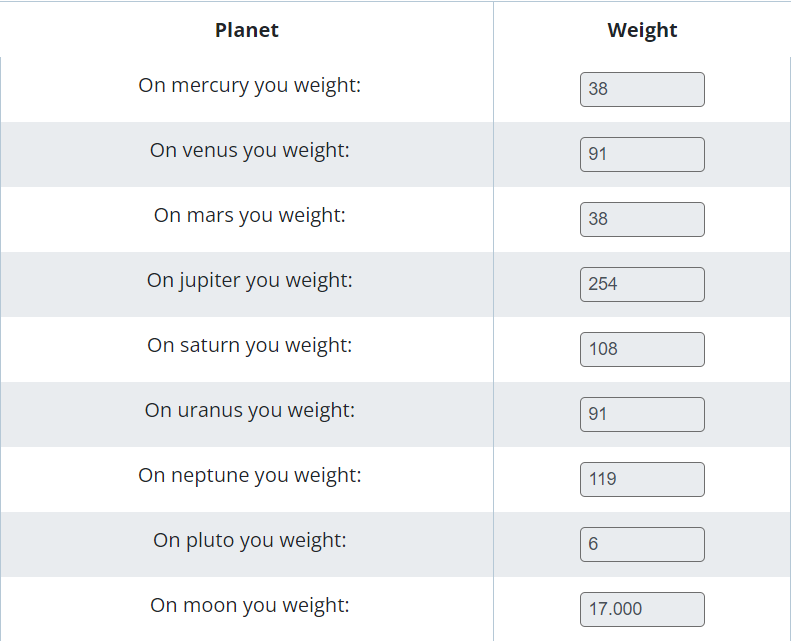# Weight on Planets Calculator

To use the weight on planets calculator, enter the weight, and press calculate button

Give Us Feedback

## Weight on Planets Calculator

Weight on Planets Calculator is issued to calculate how much you would weigh on different planets in our solar system.

## What is Weight on Planets?

Weight on planets is the study of how much an object would weigh on a particular planet, compared to its weight on Earth. The weight of an object is determined by its mass and the gravitational force acting upon it.

The gravitational force on different planets varies due to mass, size, and density differences. As a result, an object that weighs 100 pounds on Earth would weigh more or less on another planet depending on the planet's gravitational force.

For example

The weight of a 100-pound object on different planets is:### Math Tools